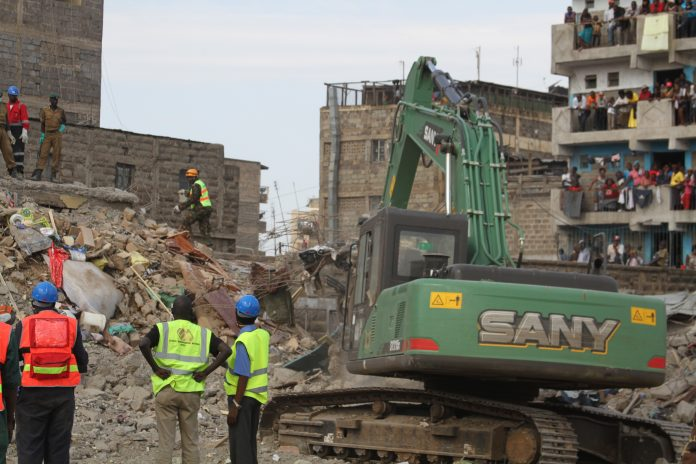By Fabrice Byebi Basema &  Nick Evans

Abstract
In a number of recent cases multi-storey buildings have collapsed when column bases have been loaded eccentrically. Usually this occurs at plot boundaries when a column in the face of the building is supported on the edge of its base and not in the centre which would require the base to project onto the neighbouring plot. This paper examines the reasons why columns fail; high ground pressures and moments on columns. It is intended to draw attention to the catastrophic consequences of eccentric loading.

When a column is located on the edge of a base designed to be centrally loaded, the ground pressure is substantially increased: the increase is shown in mathematical expressions. The base rotates as the soil under the edge is compressed creating a large moment on the column which can fail as shown in the pictures above. While engineers know that the load of the column should be supported by a beam extending from it to a penultimate column thereby loading the edge base in its centre this is sometimes ignored with fatal consequences.

Introduction
Structures fail in various ways for a number ofreasons; one of the cause is failures in structures is the incorrect design and/or construction of foundations.

The design of foundations is dependent on the geotechnical properties of the soil beneath the structure (subgrade), the magnitude of column loads to be carried and their position vis-à-vis the center of gravity of the foundation.

Often times in Kenya, engineers get the geotechnical properties of the sub-grade and the loads to be carried correct but fail to consider the eccentricity caused by the position of loads hence end up with induced moments which are not considered in their designs hence causing the structure to collapse.

This paper will highlight the various load positions, their effect on the foundation and also the pressure magnitudes that results from various eccentricity values.

Note that eccentricity is the distance from the position of the load to the center ofareaof the foundation base and that the foundation has two components; the base and the sub grade.

Analysis
In considering the influence of eccentric loading on rectangular bases three cases are considered; in the following expressions eccentricity it is shown as the variable “e”. In the first two cases the column is assumed for simplicity to have no bending strength and in the third the bending strength of the column is taken into account when locating the eccentricity.

No eccentricity; e = 0
Eccentricity is located within the middle third of bases
Eccentricity is located outside the middle third.

Case1: eccentricity e=0
In this case, there is no eccentricity hence the pressure on the ground uniform and is equal to:
P_b=V/(L×B)
Where: Pb=pressure at the base

Case2: eccentricity e ≤ L/6
The eccentricity e causes a moment which creates a non-uniform pressure on the ground so that equilibrium is maintained by the centers of action of the pressure and the load being coincident. This results in a maximum and minimum pressure at the heel and the toe of the base respectively.

These pressures are given by:
P_(min,max)=V/(B×L)∓M/Z=V/(B×L)(1∓6e/L)
M=V×e
Where: Z = Section modulus
M = Bending moment
e=Eccentricity

Case3: eccentricity (e>L/6)

In this case, the eccentricity e causes a moment hence increasing the pressure on the ground. This results in a point of zero pressure at x_o distance from the toe. The maximum pressure occurs at the heel of the base and is given by:

P_max=2V/(3B(e-Mr/V-e’-h/2))
Mr =moment of resistance
e’= column eccentricity
e=eccentricity

Note that, when the column fails, the moment strength that it was providing becomes zero and there is an increase in the pressure below the foundation base.

Discussion
These cases represent the pressure that would be felt by a substructure once loaded. As shown in the first case which consists of a center column on the foundation, only a uniformly distributed pressure is transferred to the base and felton the ground. It is the simplest and easiest to analyze and design. No moments are induced in the column in this type of foundation.

The remaining cases consist of a column eccentricity (either within the middle third of the base or not) which results in an additional moment and hence an increment in ground pressure. When the eccentricity is beyond L/6, a portion of the foundation pad will not be effective thus reducing the useful area of the base and hence resulting in a larger pressure to be felt on the ground beneath. The ineffective portion of the foundation is redundant.
In the case where the point of application of the column load is not in line with the center of area of the foundation, a moment that attempts to rotate and/or lift the foundation is created but is counteracted by the column moment of resistance. If the column is structurally sound, its Moment of Resistance pushes back the point of application of the loads closer to the center of area of the base, hence reducing and pressure exerted on the sub-grade and vice versa if the column is weak.

If the engineer designs the foundation as case one when the column is eccentric and weak foundation settlement will be unequal across the base which will create a moment in the column leading to failure and entire building collapse.

Conclusion
A common cause of foundation failure experienced by the authors is due to the incorrect assumption of the effect of the eccentricity on foundations and the type and magnitude of the pressure it creates. In checking that the allowable ground pressure is not exceeded, the pressure maybe calculated from one of the previous expressions though once the column has failed the pressure will increase further.